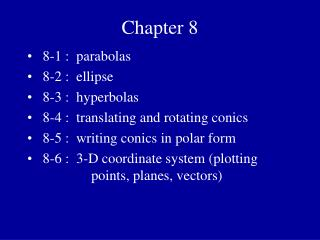DownloadDownload PresentationChapter 8

# Chapter 8

Télécharger la présentation## Chapter 8

- - - - - - - - - - - - - - - - - - - - - - - - - - - E N D - - - - - - - - - - - - - - - - - - - - - - - - - - -
##### Presentation Transcript

1. Chapter 8 • 8-1 : parabolas • 8-2 : ellipse • 8-3 : hyperbolas • 8-4 : translating and rotating conics • 8-5 : writing conics in polar form • 8-6 : 3-D coordinate system (plotting points, planes, vectors)

2. Section 8-1 • the conic sections • definition of parabola • standard form of the equation of a parabola • translating a parabola • graphing a parabola • convert from general form to standard form • reflective property of parabolas

3. Parabola: the set of all points equidistant from a particular line (the directrix) and a particular point (the focus). focus directrix

4. Parabola: the set of all points equidistant from a particular line (the directrix) and a particular point (the focus). focus directrix

5. Vertex: the vertex is midway between the focus and the directrix focus vertex directrix

6. Vertex: the vertex is midway between the focus and the directrix Focal Length: the distance from the focus to the vertex, denoted with the letter p focus p { vertex directrix

7. Standard Form of a ParabolaVertex at (0 , 0) Focus at (0 , p) 4p p p Focal length = p Focal width = Directrix at y = – p if p is negative, the graph flips over the x-axis

8. Standard Form of a ParabolaVertex at (0 , 0) p p 4p Focus at (p , 0) Focal length = p Focal width = Directrix at x = – p If the value of p is negative, the graph opens to the left (flips over the y-axis)

9. Translating a Parabola • if the parabola has a vertex of (h , k) the two equations change into: • notice that h is always with x and k is always with y • the focus and directrix will adjust accordingly

10. Sketching the Graph of a Parabola • convert the equation into standard form, if necessary • find and plot the vertex • decide which way the graph opens (based on p and which variable is squared) • add the focus and directrix to your graph • use the focal width to find two other points (these will give the parabola’s width) • graph the rest of the parabola

11. Convert Into Standard Form • to convert from general form into standard form you must use “complete the square”

12. Reflective Properties of Parabolas • if a parabola is rotated to create a 3-D version it is called a “paraboloid of revelolution” • there are many examples of parabolic reflectors in use today involving sound, light, radio and electromagnetic waves